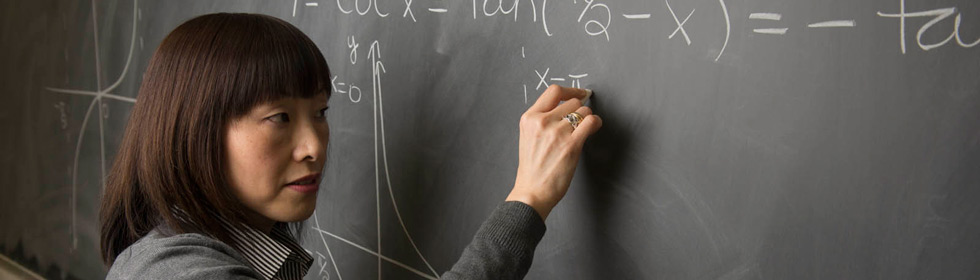Department of Mathematics & StatisticsThe Department of Mathematics & Statistics offers undergraduate degrees of Bachelor of Arts and Bachelor of Science in Mathematical Studies with concentration in:

Mathematical Studies with Secondary Education Teacher Licensure  is also available.

#### General Program Requirements

All programs require completion of the mathematics core, which consists of the following courses:

• Calculus I, II, and III (Math 150, 152, and 250)
• Introduction to Logic and Reasoning (Math 223)
• Linear Algebra (Math 321)
• Introduction to Real Analysis (Math 350)
• Computer Science 145
• Physics 151 and 151L

All students also take Math 498 and 499 (Senior Seminar and Senior Project) and complete all general education requirements. Your academic advisor will help you to design a program of study that satisfies all degree requirements.

#### Actuarial Science

According to the Society of Actuaries, the "actuary's work combines the skills of a business executive, mathematician, financial, and investment manager." It is an ideal career for those interested in studying risk, whether it is related to economics, finance, or even social issues. The U.S. Bureau of Labor Statistics anticipates a 21 percent growth rate in actuarial positions through 2018, a rate substantially higher than average.

In addition to the core requirements, a B.A. or B.S. in Mathematics with a specialization in Actuarial Science requires

 Differential Equations (Math 305) Numerical analysis (Math 465) Regression analysis (Stat 482) Stochastic models (OR 441) Finance 320 and 420 3 additional hours of finance electives Theory of interest (Math 340) Introduction to Mathematical Statistics (Stat 480a and 480b) Actuarial mathematics (Stat 486a) Economics 111 and 112 Accounting 200 and 210 6 hours of electives selected from Stat 478, Stat 485, OR 442, or Math 466

#### Applied Mathematics

Applied mathematics focuses on using mathematical theories to solve real-world problems, such as those related to structural stability, chemical interactions, or wave propagation. It encompasses the use of probability theory, numerical and data analysis, modeling, algorithm development, and simulations to explore the connections between mathematics and science and our environment. The Society for Industrial and Applied Mathematics has identified several emerging career paths for applied mathematicians, including bioinformatics, materials science, computer animation and digital imaging, finance, and climatology.

In addition to the core requirements, a B.A. or B.S. in Mathematics with a specialization in applied mathematics requires

 Differential Equations (Math 305) Complex Analysis (Math 451) Partial Differential Equations (Math 464) Numerical Analysis (Math 465) Numerical Linear Algebra (Math 466) Physics 152 and 152L 6 hours of science or engineering electives

Additionally, students take 9 hours of MATH/STAT/OR electives chosen from one of the following options:

• Math 320 and two additional courses selected from: Math 421, 437, 450, OR 440, 441, 442, Stat 480a,b
• Stat 380 and two additional courses selected from: Math 421, 437, 450, OR 440, 441, 442
• Stat 480a,b and one additional course selected from: Math 421, 437, 450, OR 440
• Math 421 and two additional courses selected from Math 437, 450, OR 440, 441, 442, Stat 480a,b

#### Pure Mathematics

This area deals with the science of structure, order, measurements, and relationships of objects or groups of objects. Those who like to problem solve are ideally suited to study pure mathematics. Since mathematics plays a vital role in in the effort to understand the world and the environment for a vast array of fields, mathematics majors have many career opportunities open to them, including careers in education, finance, technology, and engineering.

In addition to the core requirements, a B.A. or B.S. in Mathematics with a specialization in pure mathematics requires

 Introduction to Algebraic Structures (Math 320) Linear Algebra II (Math 421) Complex Analysis (Math 451) 3 hours of MATH electives at the 400-level Abstract Algebra (Math 420) Real Analysis (Math 450) Either Math 435 (Euclidean and Non-Euclidean Geometry) or 437 (Differential Geometry) 12 hours of mathematics, statistics, operations research, courses from the School of Engineering, biology, chemistry, or physics at the 200 level or above

#### Mathematical Studies - Secondary Education Teacher Licensure

Students who desire to teach youth and who are able to generate excitement and understand of mathematics as well as impart its relevance to real-life applications are ideal candidates to major in mathematics for secondary education teacher certification. They are the largest group of mathematics majors at SIUE. The Department has developed strong relationships with local high schools and consults regularly about ways to enhance teacher training opportunities. Because math is a part of all schools' core curriculum, the outlook for students majoring in mathematical studies with secondary education licensure is strong.

In addition to the core requirements, a B.A. or B.S. in Mathematical Studies with Secondary Education teacher licensure requires

 Teaching of Secondary Mathematics (Math 311) Development of Modern Mathematics (Math 400) Statistics for Applications (Stat 380) Methods and Practical experience (CI 315a,b, CI352) The Exceptional Child (SPE 400) Adolescent Literacy (CI 440) Introduction to Algebraic Structures (Math 320) Foundations for Euclidean and Non-Euclidean Geometry (Math 435) Introduction to Education (CI 200) Educational Psychology and Foundations of Multicultural Education (EPFR 315, 320) Either Math 305 and one 400 level MATH/STAT/OR elective or two 400 level MATH/STAT/OR electives